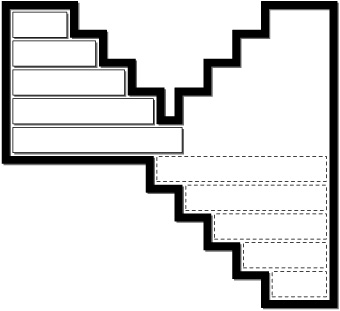# Homework 5

#### Problem 1.

A directed graph is strongly connected if for every pair of vertices $$u$$, $$v$$, there is a path from $$u$$ to $$v$$.

Show that testing whether a given directed graph is strongly connected is in NL. Then show that it is NL-complete by giving a log-space reduction from Reachability to StrongConnectedness.

#### Problem 2.

The problem AcyclicGraph takes as input a directed graph and answers yes if the graph does not have a directed cycle.

Show that AcyclicGraph is NL-complete.

Hint: What is the negation of this problem? Use the Immermann-Szelepcsényi Theorem.

#### Problem 3.

Consider a sliding puzzle, like this one, generalized to $$n$$ blocks:To be more precise, the frame of the puzzle is a rectilinear polygon (a polygon with horizontal and vertical edges only), all vertices of which are integers of polynomial size. There are $$n$$ sliding blocks, which are rectangles of integer side length contained inside the puzzle frame. We are given an initial position of each block, and a target position for at least one of the blocks. A move means to translate a block horizontally or vertically (as far as you want) without intersecting another block or the frame (touching is allowed).

Show that there is a PSPACE program that prints out the shortest sequence of moves from the initial configuration to a target configuration.

Note that the length of the shortest path could be exponential, so we cannot store it. Instead assume that your program can print out a move whenever it wishes to. Of course the moves have to be printed in the correct order.